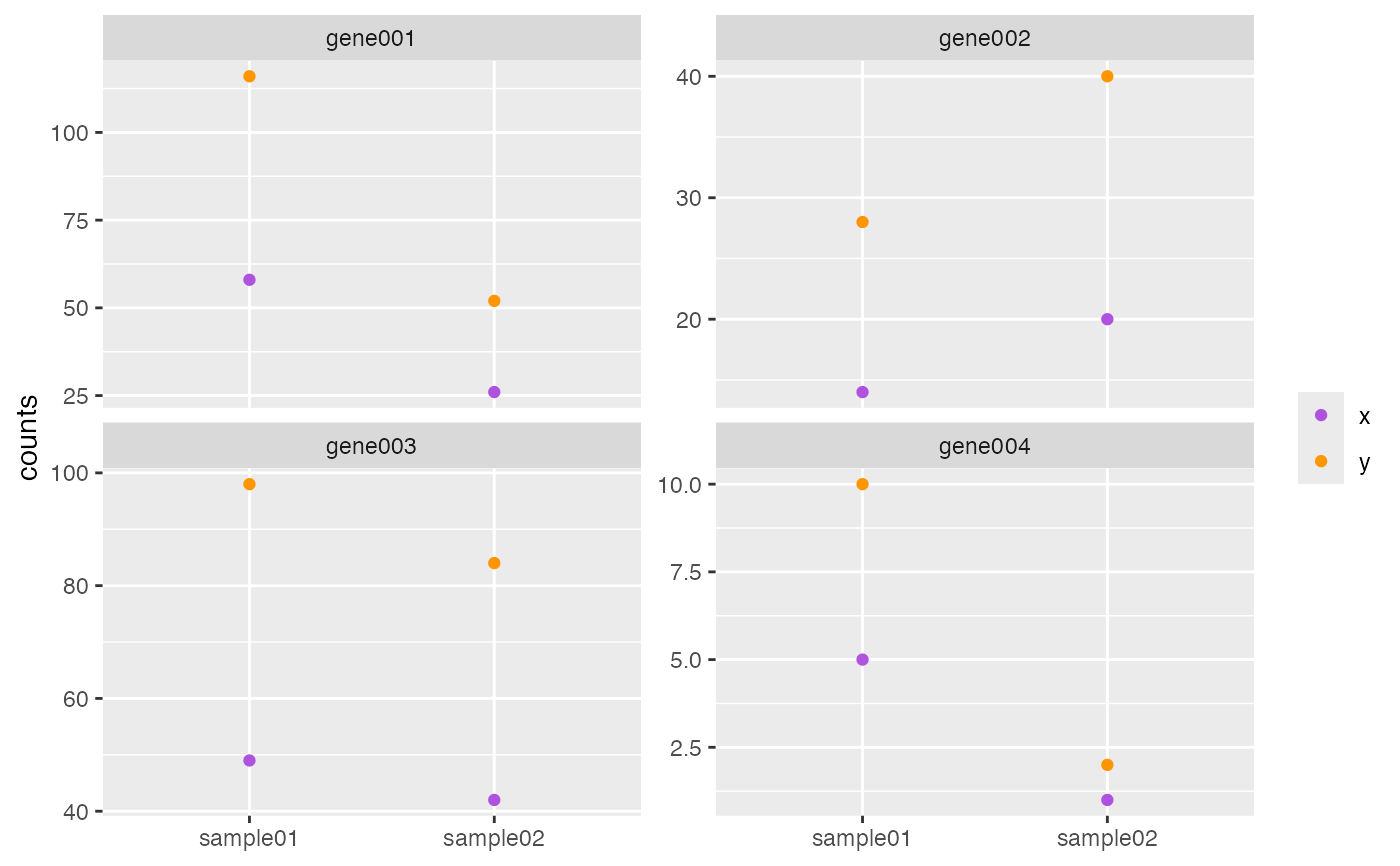Compare feature expression correlation across two data sets.

## Usage

plotCountsCorrelation(x, y, ...)

# S4 method for matrix,matrix
plotCountsCorrelation(
x,
y,
i = NULL,
j = NULL,
xName = "x",
yName = "y",
labels = list(title = NULL, subtitle = NULL, color = NULL, x = NULL, y = "counts")
)

## Arguments

x

Object.

y

Object.

i

Indices specifying elements to extract or replace. Indices are numeric or character vectors, empty (missing), or NULL.

help(topic = "Extract", package = "base")

j

Indices specifying elements to extract or replace. Indices are numeric or character vectors, empty (missing), or NULL.

help(topic = "Extract", package = "base")

xName, yName

character(1). Color legend name for value in x or y.

labels

list. ggplot2 labels. See ggplot2::labs() for details.

...

## Value

Graphical output.

## Note

Updated 2023-10-05.

## Examples

data(RangedSummarizedExperiment, package = "AcidTest")

## matrix ====
x <- SummarizedExperiment::assay(RangedSummarizedExperiment)
x <- x[seq_len(4L), seq_len(2L)]
y <- x * 2L
plotCountsCorrelation(x, y)